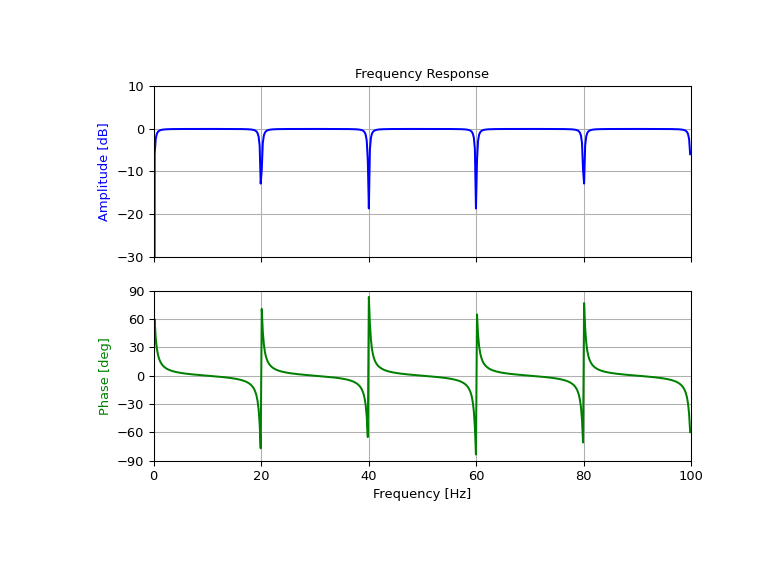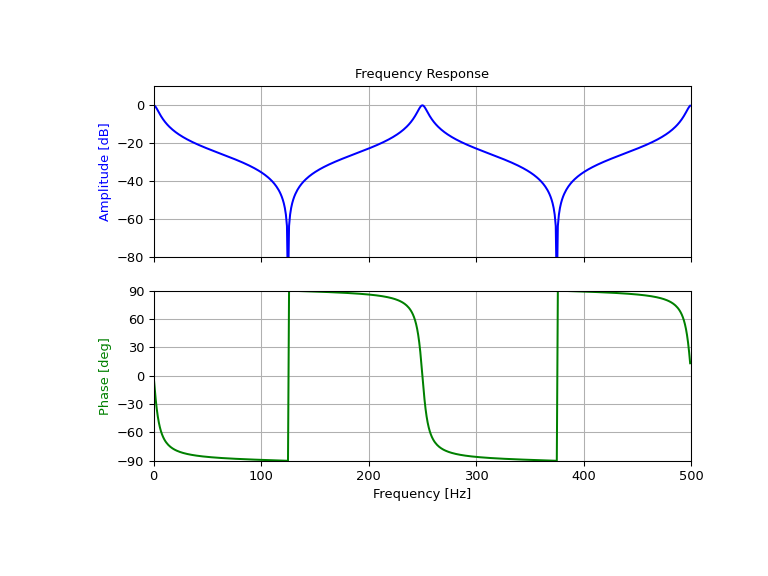# scipy.signal.iircomb¶

scipy.signal.iircomb(w0, Q, ftype='notch', fs=2.0)[source]

Design IIR notching or peaking digital comb filter.

A notching comb filter is a band-stop filter with a narrow bandwidth (high quality factor). It rejects a narrow frequency band and leaves the rest of the spectrum little changed.

A peaking comb filter is a band-pass filter with a narrow bandwidth (high quality factor). It rejects components outside a narrow frequency band.

Parameters
w0float

Frequency to attenuate (notching) or boost (peaking). If fs is specified, this is in the same units as fs. By default, it is a normalized scalar that must satisfy `0 < w0 < 1`, with `w0 = 1` corresponding to half of the sampling frequency.

Qfloat

Quality factor. Dimensionless parameter that characterizes notch filter -3 dB bandwidth `bw` relative to its center frequency, `Q = w0/bw`.

ftype{‘notch’, ‘peak’}

The type of comb filter generated by the function. If ‘notch’, then it returns a filter with notches at frequencies `0`, `w0`, `2 * w0`, etc. If ‘peak’, then it returns a filter with peaks at frequencies `0.5 * w0`, `1.5 * w0`, `2.5 * w0``, etc. Default is ‘notch’.

fsfloat, optional

The sampling frequency of the signal. Default is 2.0.

Returns
b, andarray, ndarray

Numerator (`b`) and denominator (`a`) polynomials of the IIR filter.

Raises
ValueError

If w0 is less than or equal to 0 or greater than or equal to `fs/2`, if fs is not divisible by w0, if ftype is not ‘notch’ or ‘peak’

Notes

For implementation details, see . The TF implementation of the comb filter is numerically stable even at higher orders due to the use of a single repeated pole, which won’t suffer from precision loss.

References

1

Sophocles J. Orfanidis, “Introduction To Signal Processing”, Prentice-Hall, 1996

Examples

Design and plot notching comb filter at 20 Hz for a signal sampled at 200 Hz, using quality factor Q = 30

```>>> from scipy import signal
>>> import matplotlib.pyplot as plt
```
```>>> fs = 200.0  # Sample frequency (Hz)
>>> f0 = 20.0  # Frequency to be removed from signal (Hz)
>>> Q = 30.0  # Quality factor
>>> # Design notching comb filter
>>> b, a = signal.iircomb(f0, Q, ftype='notch', fs=fs)
```
```>>> # Frequency response
>>> freq, h = signal.freqz(b, a, fs=fs)
>>> response = abs(h)
>>> # To avoid divide by zero when graphing
>>> response[response == 0] = 1e-20
>>> # Plot
>>> fig, ax = plt.subplots(2, 1, figsize=(8, 6))
>>> ax.plot(freq, 20*np.log10(abs(response)), color='blue')
>>> ax.set_title("Frequency Response")
>>> ax.set_ylabel("Amplitude (dB)", color='blue')
>>> ax.set_xlim([0, 100])
>>> ax.set_ylim([-30, 10])
>>> ax.grid()
>>> ax.plot(freq, np.unwrap(np.angle(h))*180/np.pi, color='green')
>>> ax.set_ylabel("Angle (degrees)", color='green')
>>> ax.set_xlabel("Frequency (Hz)")
>>> ax.set_xlim([0, 100])
>>> ax.set_yticks([-90, -60, -30, 0, 30, 60, 90])
>>> ax.set_ylim([-90, 90])
>>> ax.grid()
>>> plt.show()
```Design and plot peaking comb filter at 250 Hz for a signal sampled at 1000 Hz, using quality factor Q = 30

```>>> fs = 1000.0  # Sample frequency (Hz)
>>> f0 = 250.0  # Frequency to be retained (Hz)
>>> Q = 30.0  # Quality factor
>>> # Design peaking filter
>>> b, a = signal.iircomb(f0, Q, ftype='peak', fs=fs)
```
```>>> # Frequency response
>>> freq, h = signal.freqz(b, a, fs=fs)
>>> response = abs(h)
>>> # To avoid divide by zero when graphing
>>> response[response == 0] = 1e-20
>>> # Plot
>>> fig, ax = plt.subplots(2, 1, figsize=(8, 6))
>>> ax.plot(freq, 20*np.log10(np.maximum(abs(h), 1e-5)), color='blue')
>>> ax.set_title("Frequency Response")
>>> ax.set_ylabel("Amplitude (dB)", color='blue')
>>> ax.set_xlim([0, 500])
>>> ax.set_ylim([-80, 10])
>>> ax.grid()
>>> ax.plot(freq, np.unwrap(np.angle(h))*180/np.pi, color='green')
>>> ax.set_ylabel("Angle (degrees)", color='green')
>>> ax.set_xlabel("Frequency (Hz)")
>>> ax.set_xlim([0, 500])
>>> ax.set_yticks([-90, -60, -30, 0, 30, 60, 90])
>>> ax.set_ylim([-90, 90])
>>> ax.grid()
>>> plt.show()
```